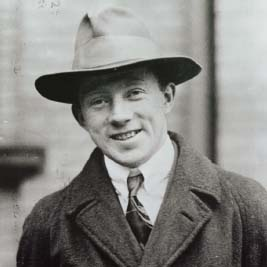NextPrevious

# How do quantum physicists regard light waves?

Added to the mix of the mathematically rich quantum theory was an idea developed by French physicist Prince Louis Victor Pierre Raymond de Broglie (1892–1987), who discovered the wave nature of electrons and of particles in general (and also devised a mathematical explanation of the kinetic theory of heat). He determined that not only do light waves often exhibit particle-like properties, but particles also often exhibit wave-like properties.

This opened a can of quantum worms. From there, two different formulations of quantum mechanics developed: first, the wave mechanics of Austrian physicist Erwin Schrödinger (1887–1961), who used a mathematical entity (the wave function) related to the probability of finding a particle in space at a given point. (Schrödinger also developed a model of the atom that differed from the traditional Niels Bohr model); second and mathematically equal to Schrödinger’s theory was the matrix mechanics of German physicist Werner Karl Heisenberg (1901-1976; for more about Heisenberg, see below).German physicist Werner Karl Heisenberg developed the uncertainty principal, which states that it is impossible to note both the exact position and exact motion of an object at the same time.

Close

This is a web preview of the "The Handy Math Answer Book" app. Many features only work on your mobile device. If you like what you see, we hope you will consider buying. Get the App